Function Repository Resource:

# BinormalVector

Compute the binormal vector of a curve

Contributed by: Wolfram Staff (original content by Alfred Gray)
 ResourceFunction["BinormalVector"][c,t] computes the binormal vector of curve c parametrized by t.

## Details and Options

The binormal vector is the cross product of the tangent vector and the normal vector.

## Examples

### Basic Examples (2)

Consider the curve:

 In:=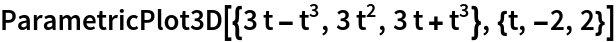Out=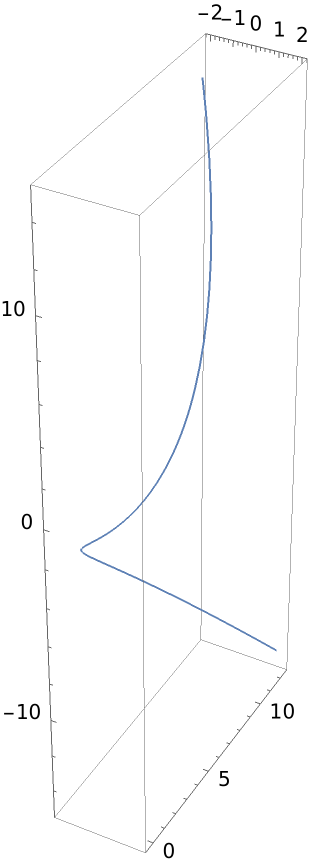The binormal vector:

 In:=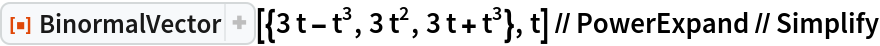Out=### Scope (2)

Use a helix:

 In:=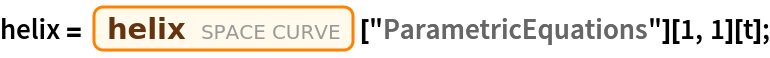The binormal vector:

 In:=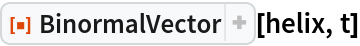Out=Calculate the normal vector using the resource function NormalVector:

 In:=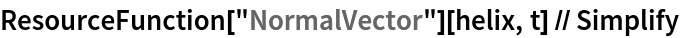Out=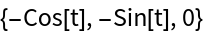The normal vector is the cross product of the binormal vector and the tangent vector. Check this using the previous computation along with the resource function TangentVector:

 In:=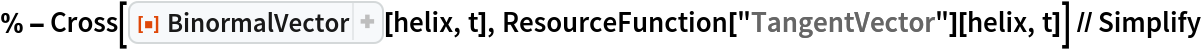Out=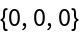The binormal surface associated to a curve is generated by its binormal vector field. It can be computed with the resource function BinormalSurface:

 In:=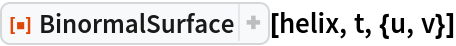Out=Check this:

 In:=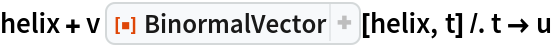Out=Combine the binormal surface with the helix curve:

 In:=Out=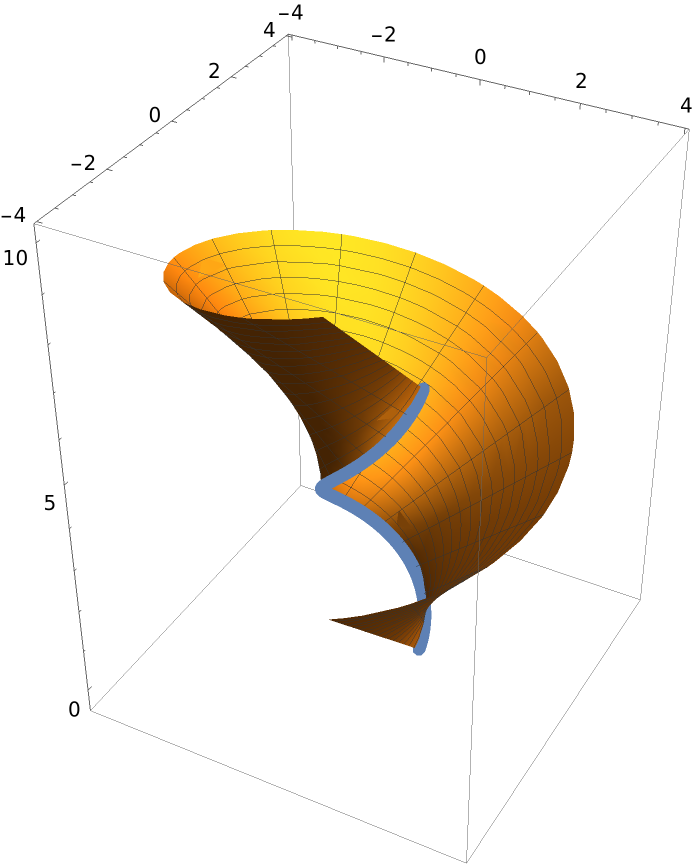### Properties and Relations (7)

Define a unit speed helix:

 In:=Its binormal vector:

 In:=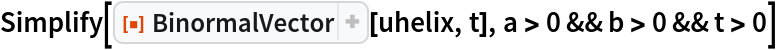Out=The derivative of the binormal vector:

 In:=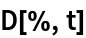Out=The torsion, via the resource function CurveTorsion:

 In:=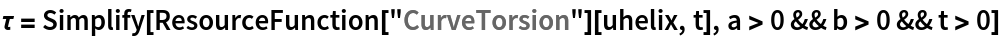Out=The normal vector, via the resource function NormalVector:

 In:=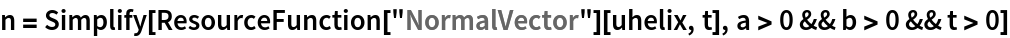Out=The torsion multiplied by the normal is the derivative of the binormal:

 In:=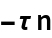Out=Using FrenetSerretSystem, the binormal vector is the last element of the second List:

 In:=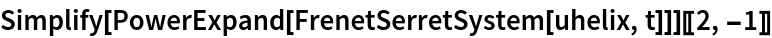Out=Enrique Zeleny

## Version History

• 2.0.0 – 30 April 2020
• 1.0.0 – 28 April 2020